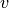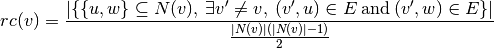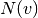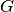NetworkX

#### Previous topic

average_clustering

#### Next topic

closeness_centrality

# node_redundancy¶

node_redundancy(G, nodes=None)[source]

Compute bipartite node redundancy coefficient.

The redundancy coefficient of a nodeis the fraction of pairs of neighbors ofthat are both linked to other nodes. In a one-mode projection these nodes would be linked together even ifwere not there.whereare the neighbors ofin.

Parameters : G : graph A bipartite graph nodes : list or iterable (optional) Compute redundancy for these nodes. The default is all nodes in G. redundancy : dictionary A dictionary keyed by node with the node redundancy value.

References

 [R126] Latapy, Matthieu, Clémence Magnien, and Nathalie Del Vecchio (2008). Basic notions for the analysis of large two-mode networks. Social Networks 30(1), 31–48.

Examples

>>> from networkx.algorithms import bipartite
>>> G = nx.cycle_graph(4)
>>> rc = bipartite.node_redundancy(G)
>>> rc
1.0


Compute the average redundancy for the graph:

>>> sum(rc.values())/len(G)
1.0


Compute the average redundancy for a set of nodes:

>>> nodes = [0, 2]
>>> sum(rc[n] for n in nodes)/len(nodes)
1.0